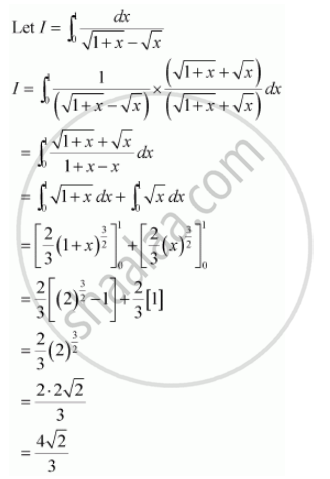Share

# Evaluate the Definite Integrals Int_0^1 Dx/(Sqrt(1+X) - Sqrtx) - CBSE (Science) Class 12 - Mathematics

ConceptDefinite Integral as the Limit of a Sum

#### Question

Evaluate the definite integrals int_0^1 dx/(sqrt(1+x) - sqrtx)

#### SolutionIs there an error in this question or solution?

#### Video TutorialsVIEW ALL 

Solution Evaluate the Definite Integrals Int_0^1 Dx/(Sqrt(1+X) - Sqrtx) Concept: Definite Integral as the Limit of a Sum.
S